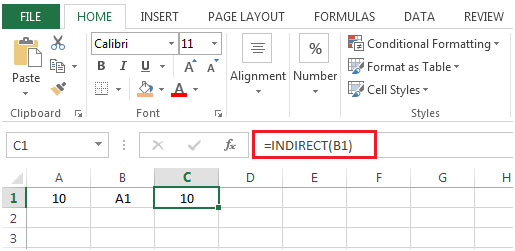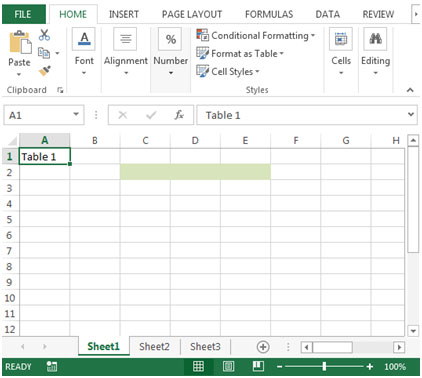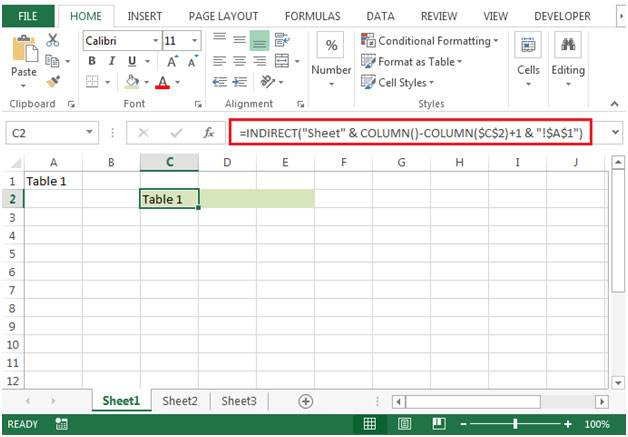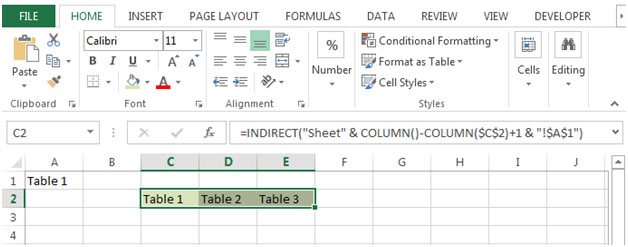# Retrieving a Specific Cell from Multiple Sheets in Excel 2010

While preparing a report, if we want to copy the contents of a specific cell from all the sheets in a specific row then we can use a combination of INDIRECT & COLUMN functions.

INDIRECT: Returns the reference specified by a text string.

Syntax: =INDIRECT(ref_text,A1)

ref_text is a reference to a cell. If ref_text is not a valid cell reference then INDIRECT function will return #REF error.

The second argument A1 refers to a logical value that specifies type of reference is contained in the cell ref_text.

If a1 is TRUE or omitted then ref_text is determined as A1-style reference.

If a1 is FALSE then ref_text is determined as R1C1 style reference.
Example: If value in cell A1 contains 10, B1 contains A1 & we use INDIRECT function in cell C1=INDIRECT(B1), then result would be 10COLUMN: Returns the column number of a reference.

Syntax: =COLUMN(reference)

Reference: It is a reference to a cell or range of cells.
Let us take an example:

• We have three sheets in the workbook.
• Cell A1 contains specific text in all the sheets.• We want a formula that will copy the contents from all the sheets in the current sheet.
• The formula we will use in cell C2would be
=INDIRECT("Sheet" & COLUMN()-COLUMN(\$C\$2)+1 & "!\$A\$1")
• Press Enter on your keyboard.• Copying the formula to cell D2 & E2, we will get the value of cell A1 from the respective sheets.In this way we will find a formula very helpful that will give a value from all the multiple sheets in the workbook.

1.What should we do when we need to have values from upto 400 sheets in a single column?

•Using VBA is the best solution I can think of.

2.that was great, but when i am changing sheet names it is not working

Terms and Conditions of use

The applications/code on this site are distributed as is and without warranties or liability. In no event shall the owner of the copyrights, or the authors of the applications/code be liable for any loss of profit, any problems or any damage resulting from the use or evaluation of the applications/code.